## Method

Lidar-Monocular Visual Odometry 2 [la] [LIMO2]
https://github.com/johannes-graeter/limo

Submitted on 26 Jul. 2018 12:57 by
Johannes Gräter (Institute of Measurement and Control, Karlsruhe Initute of Technology)

 Running time: 0.2 s Environment: 2 cores @ 2.5 Ghz (C/C++)

 Method Description: This is a follow up of LIMO: * Use 5-point algorithm and pose only adjustment for prior. * Minor changes in Landmark Selection Parameters: See Paper Latex Bibtex: @article{graeter2018limo, title={LIMO: Lidar-Monocular Visual Odometry}, author={Graeter, Johannes and Wilczynski, Alexander and Lauer, Martin}, journal={arXiv preprint arXiv:1807.07524}, year={2018} }

## Detailed Results

From all test sequences (sequences 11-21), our benchmark computes translational and rotational errors for all possible subsequences of length (5,10,50,100,150,...,400) meters. Our evaluation ranks methods according to the average of those values, where errors are measured in percent (for translation) and in degrees per meter (for rotation). Details for different trajectory lengths and driving speeds can be found in the plots underneath. Furthermore, the first 5 test trajectories and error plots are shown below.

## Test Set Average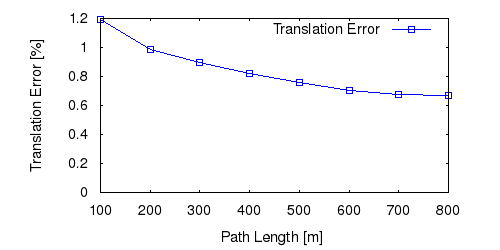This figure as: png eps pdf txt gnuplot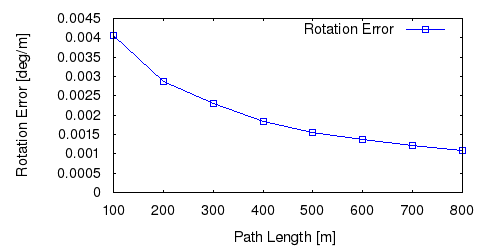This figure as: png eps pdf txt gnuplot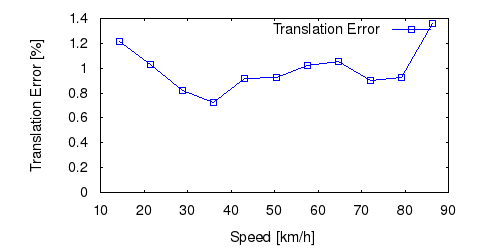This figure as: png eps pdf txt gnuplot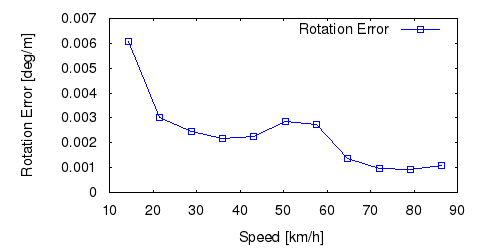This figure as: png eps pdf txt gnuplot

## Sequence 11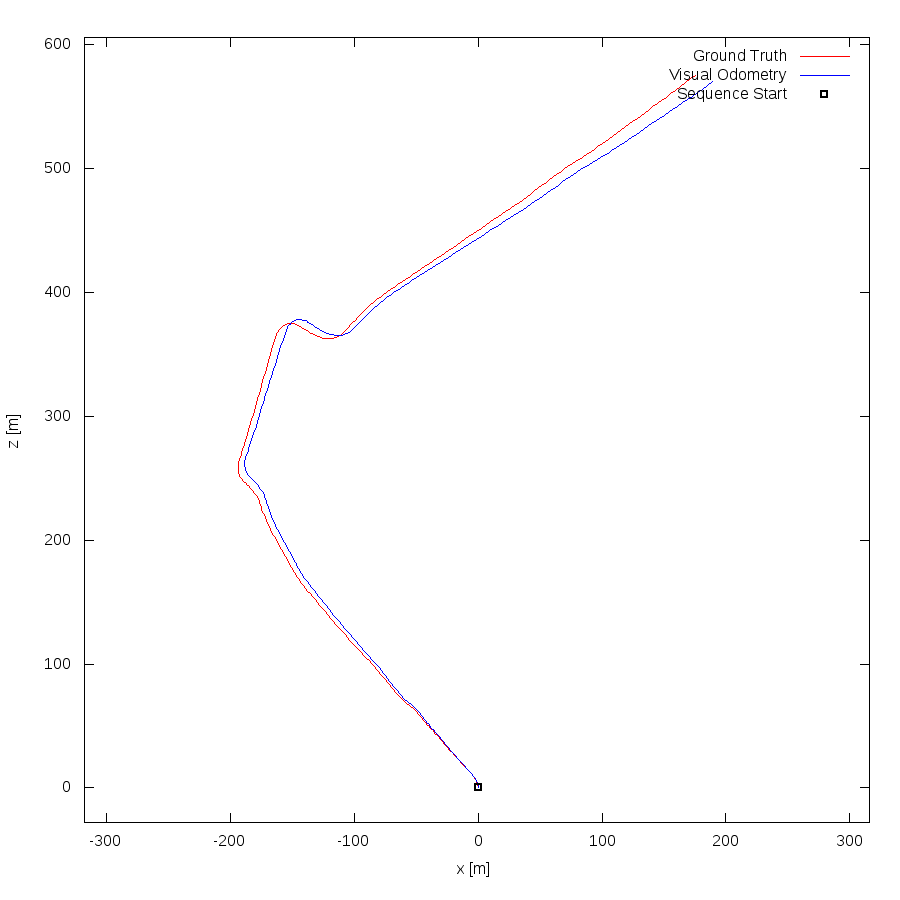This figure as: png eps pdf txt gnuplot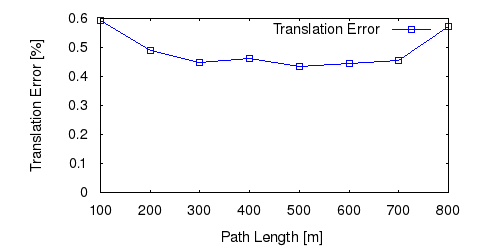This figure as: png eps pdf txt gnuplot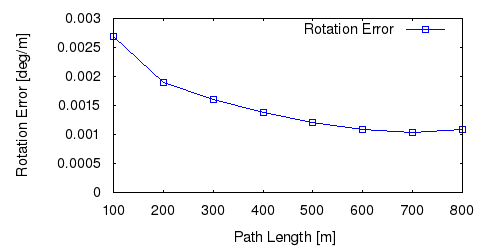This figure as: png eps pdf txt gnuplotThis figure as: png eps pdf txt gnuplotThis figure as: png eps pdf txt gnuplot

## Sequence 12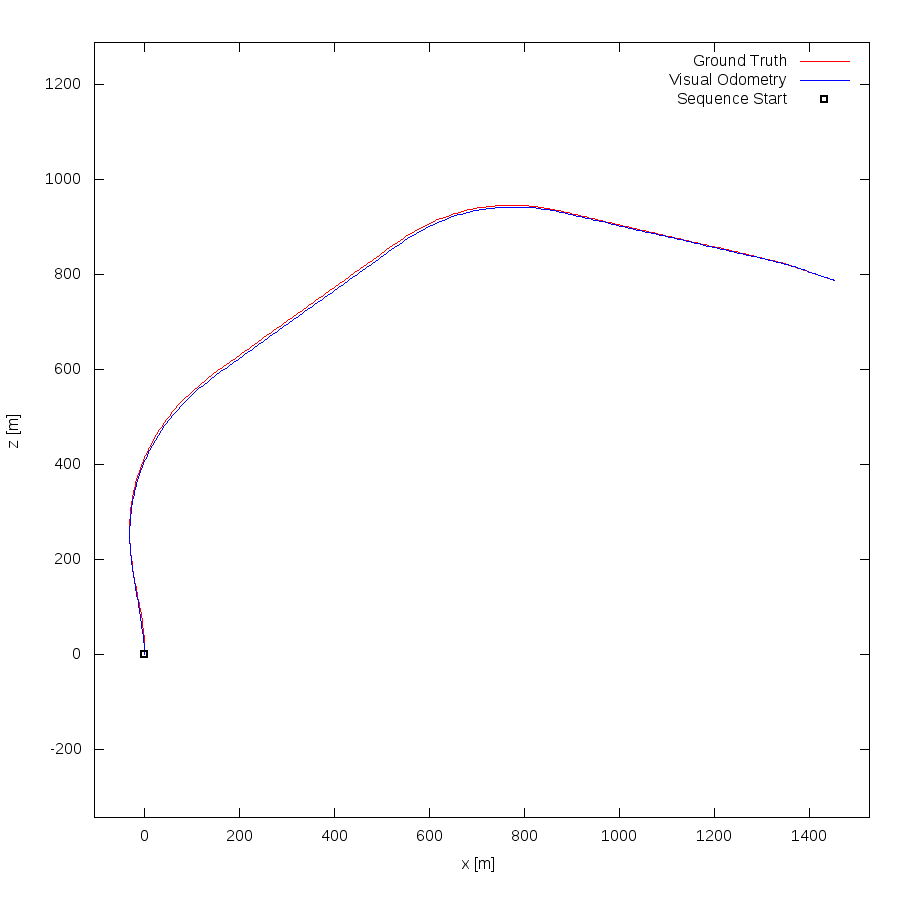This figure as: png eps pdf txt gnuplot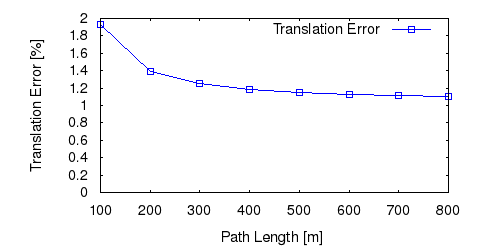This figure as: png eps pdf txt gnuplot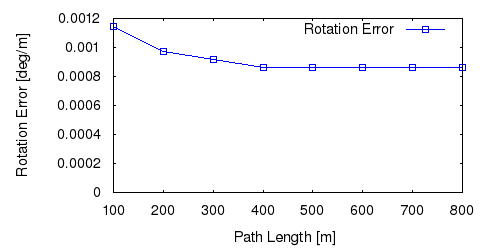This figure as: png eps pdf txt gnuplot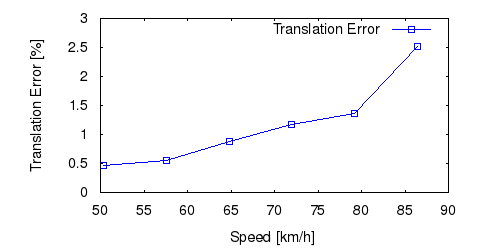This figure as: png eps pdf txt gnuplot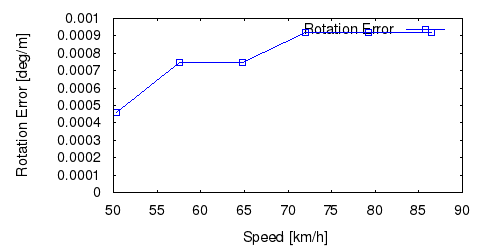This figure as: png eps pdf txt gnuplot

## Sequence 13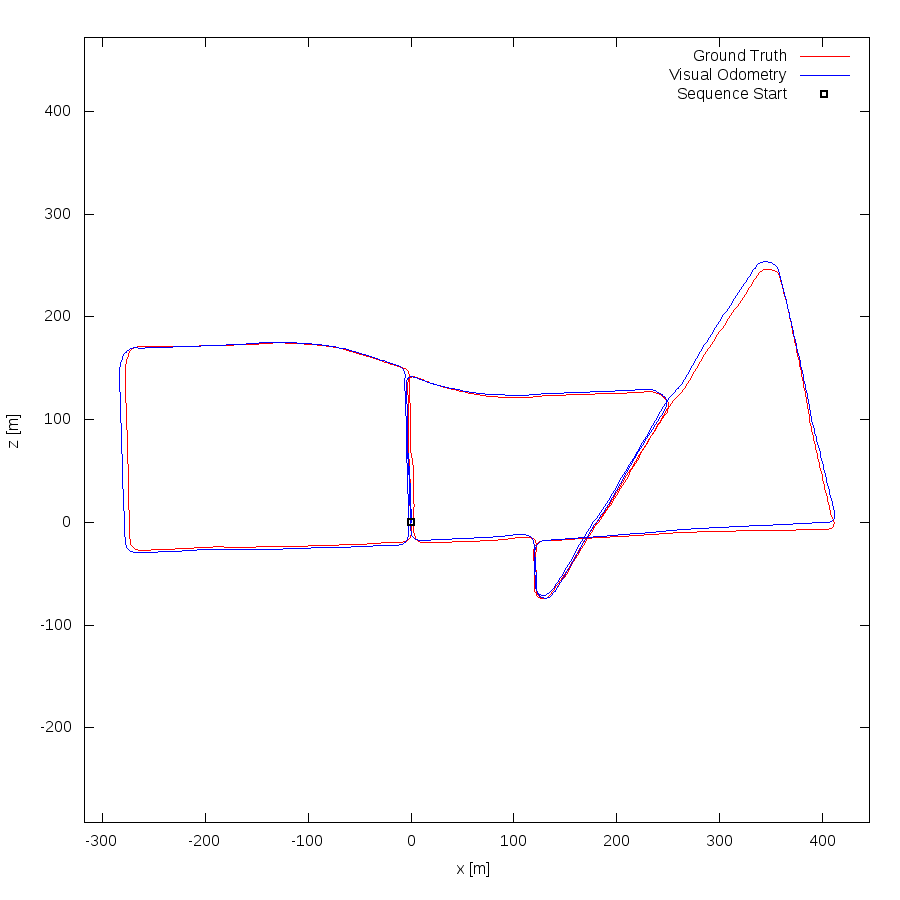This figure as: png eps pdf txt gnuplotThis figure as: png eps pdf txt gnuplot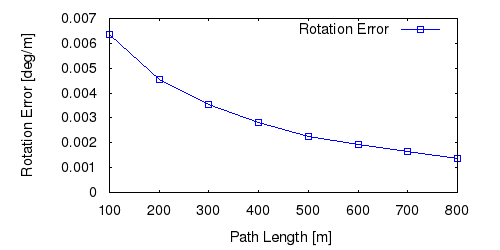This figure as: png eps pdf txt gnuplot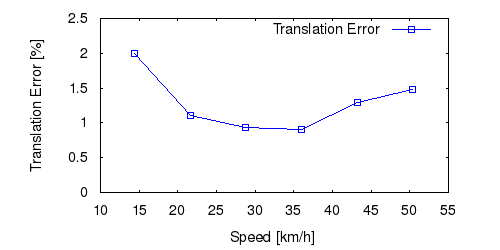This figure as: png eps pdf txt gnuplot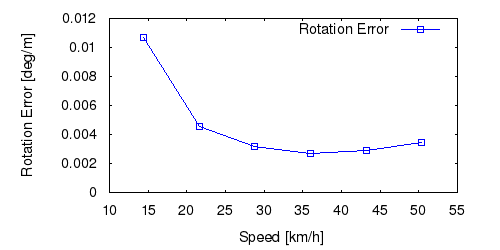This figure as: png eps pdf txt gnuplot

## Sequence 14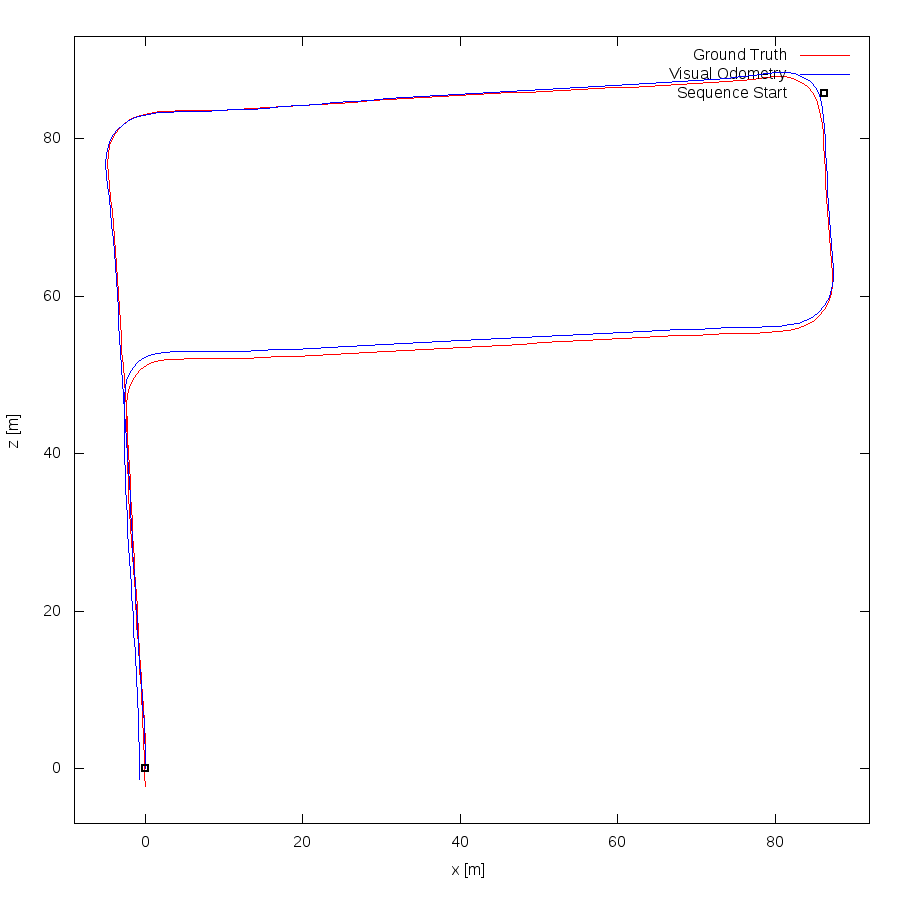This figure as: png eps pdf txt gnuplot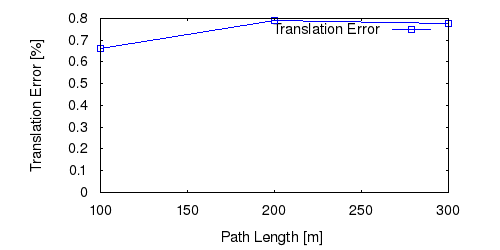This figure as: png eps pdf txt gnuplot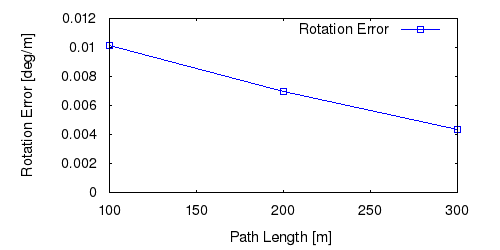This figure as: png eps pdf txt gnuplotThis figure as: png eps pdf txt gnuplotThis figure as: png eps pdf txt gnuplot

## Sequence 15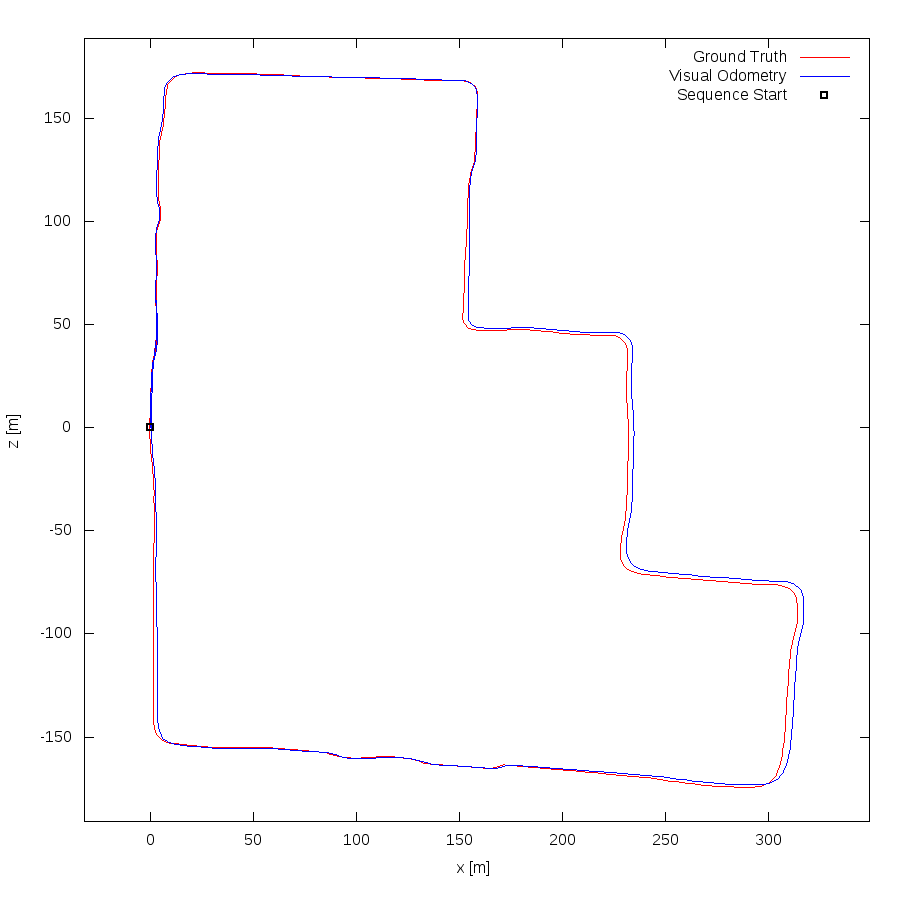This figure as: png eps pdf txt gnuplot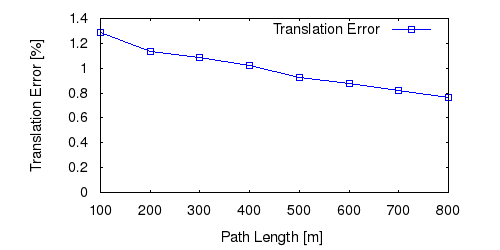This figure as: png eps pdf txt gnuplot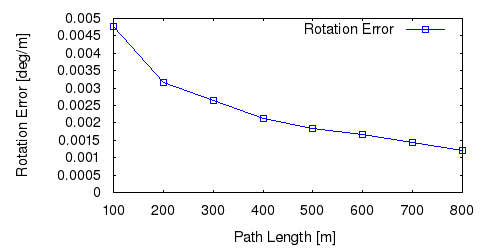This figure as: png eps pdf txt gnuplot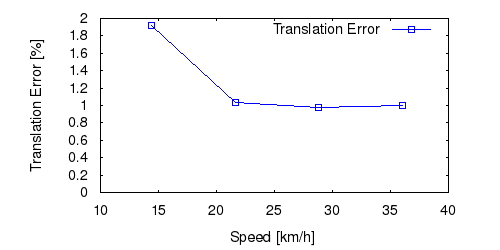This figure as: png eps pdf txt gnuplot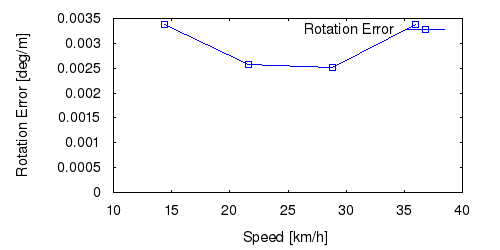This figure as: png eps pdf txt gnuplot